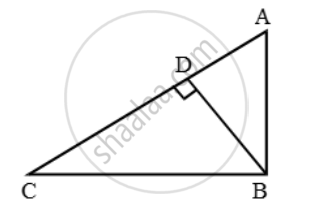Share

# If a Perpendicular is Drawn from the Vertex Containing the Right Angle of a Right Triangle to the Hypotenuse Then Prove that the Triangle on Each Side of the Perpendicular Are Similar to Each Other - CBSE Class 10 - Mathematics

ConceptSimilarity Examples and Solutions

#### Question

If a perpendicular is drawn from the vertex containing the right angle of a right triangle to the hypotenuse then prove that the triangle on each side of the perpendicular are similar to each other and to the original triangle. Also, prove that the square of the perpendicular is equal to the product of the lengths of the two parts of the hypotenuse

#### Solution

Given: A right triangle ABC right angled at B, BD ⊥ AC.

To Prove:

(iii) ∆BDC ~ ∆ABC             (iv) BD2 = AD × DC

(v) AB2 = AD × AC         (vi) BC2 = CD × AC

Proof:(i) We have,

∠ABD + ∠DBC = 90º

Also, ∠C + ∠DBC + ∠BDC = 180º

⇒ ∠C + ∠DBC + 90º = 180º

⇒ ∠C + ∠DBC = 90º

But, ∠ABD + ∠DBC = 90º

∴ ∠ABD + ∠DBC = ∠C + ∠DBC

⇒ ∠ABD = ∠C ….(i)

Thus, in ∆ADB and ∆BDC, we have ∠ABD = ∠C [From (i)] and, ∠ADB = ∠BDC [Each equal to 90º]

So, by AA-similarity criterion, we have

(ii) In ∆ADB and ∆ABC, we have

∠ADB = ∠ABC [Each equal to 90º]   and, ∠A = ∠A [Common]

So, by AA-similarity criterion, we have

(iii)In ∆BDC and ∆ABC, we have

∠BDC = ∠ABC [Each equal to 90º]

∠C = ∠C [Common]

So, by AA-similarity criterion, we have

∆BDC ~ ∆ABC

(iv) From (i), we have

\Rightarrow \frac{AD}{BD}=\frac{BD}{DC}

⇒ BD^2 = AD × DC

(v) From (ii), we have

\Rightarrow \frac{AD}{AB}=\frac{AB}{AC}

⇒ AB2 = AD × AC

(vi) From (iii), we have

\Rightarrow \frac{BC}{AC}=\frac{DC}{BC}

∆BDC ~ ∆ABC

⇒ BC2 = CD × AC

Is there an error in this question or solution?

#### Video TutorialsVIEW ALL 

Solution If a Perpendicular is Drawn from the Vertex Containing the Right Angle of a Right Triangle to the Hypotenuse Then Prove that the Triangle on Each Side of the Perpendicular Are Similar to Each Other Concept: Similarity Examples and Solutions.
S